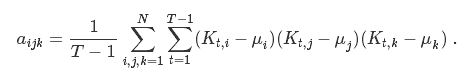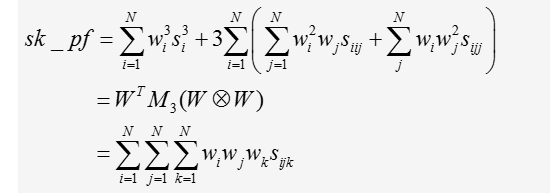## Computing the Co-Skewness matrix of portfolio

Dear all,

I want to compute the skewness of portfolio. By reading loads of literature, I found that the formula of computing the skewness of portfolio is as following:

skewness_p=ω' M3 (ωω)

where M3=E[(r-μ) (r-μ)'(r-μ)']={aijk}

the denote the kronecker product, M3 is the co-skewness matrix, and r and u are the return matrix and average return matrix, respectively.

However, I don't know how to decompose the expectation of the co-skewness matrix M3, thus I found a alternative way to calculate the M3, that's:

The co-skewness matrix of M3 of dimensions (N,N2) can be represented by N Aijk matrixes (N,N) such that:
M3=[ A1jk A1jk ... ANjk ]

where j,k=1,…,N as well as for an index i. The individual elements of the matrix can be obtained as:the capital K denote the element in the TxN return matrix, T is the number of observations and N is the number of assets.

According to the formula, I write down the following code:

proc iml;

T=j(81,100,.); /* This is my return matrix, for example*/

W=j(1,100,.); /* This is my weights matrix, for example*/

call randgen(T,'normal');

call randgen(W,'normal');

n=ncol(T);

r=nrow(T);

M=j(n,n,.);

do i=1 to n;

S=j(n,n,.);

do j=1 to n;

do k=1 to n;

u=0;

do y=1 to r-1;

u=u+((T[y,i]-T[:,i])*(T[y,j]-T[:,j])*(T[y,k]-T[:,k]));

end;

s[j,k]=u/(y-1);

end;

end;

M=M||S;

end;

M3=M[,(n+1):(n*n+n)];

skew=W*M3*(W@W);

print skew ;

quit;

My problem comes out...There are 4 loops in this piece of code, and the speed is extremely low! I made a few simulation to approximate the time elapse. If I have 20 assets(n=20) in my portfolio, it will cost me 8 seconds, if I have 100 assets in my portfolio, it will cost me around 4 minutes. If I have 200 asset in my portfolio, it costs me 21 minutes! For the number of assets equal to 300, I run approximately 60 minutes and it still hasn't finished, so I have to abort it...The problem becomes unmanageable, I have 185,000 portfolios need to  be evaluated, and around 17000 of them have more than 200 assets(n>=200). Within these 17000 portfolio, there are even more than 1200 portfolios with more than 1000 assets!  This problem really makes me desperate! Does anyone know how can I improve this code? I reaaaaally reaaaally appreciate!!!

1 ACCEPTED SOLUTION

Accepted Solutions

## Re: Computing the Co-Skewness matrix of portfolio

I think the key is to think of the "centered" matrix X = T[1:(r-1),] - mean(T);

In terms of that matrix, the double loops over j and k become essentially an XX computation, except that you have to include the ith column as a weight matrix.  That means that you can replace the j and k loops by X*diag(X[, i])*X / (r-1);

The resulting code ought to be lightening fast.

10 REPLIES 10

## Re: Computing the Co-Skewness matrix of portfolio

I see that you are hedging your bets by cross-posting this question to multiple forums: Computing the Co-Skewness matrix of portfolio - MATLAB Answers - MATLAB Central

I'm pretty sure that the four loops are vectorizable, but that is not the only performance issue here. You are also concatenating inside a loop, which is inefficient.See Pre-allocate arrays to improve efficiency - The DO Loop.  So while you contemplate how to vectorize the summation computations, start by allocating the M3 matrix outside of the loop and then filling each column within the loop:

M3 = j(n, n*n);
begCol = 1;
endCol = n;
do i=1 to n;
S=j(n,n,.);
do j=1 to n;
do k=1 to n;
u=0;
do y=1 to r-1;
u=u+((T[y,i]-T[:,i])*(T[y,j]-T[:,j])*(T[y,k]-T[:,k]));
end;
s[j,k]=u/(y-1);
end;
end;
M3[,begCol:endCol]=S;
begCol=endCol+1;
endCol=endCol+n;
end;
skew=W*M3*(W@W);
print skew ;

## Re: Computing the Co-Skewness matrix of portfolio

The inner loop is just the sum of the elementwise product of the centered ith, jth, and kth columns (not including the last row):

M3 = j(n, n*n);

begCol = 1;

endCol = n;

mean = mean(T);

idx = 1:(r-1);

do i=1 to n;

Ti = T[idx, i] - mean;

S=j(n,n,.);

do j=1 to n;

Tj = T[idx, j] - mean;

do k=1 to n;

Tk = T[idx, k] - mean;

s[j,k]= sum( Ti#Tj#Tk ) / (r-1);

end;

end;

M3[,begCol:endCol]=S;

begCol=endCol+1;

endCol=endCol+n;

end;

skew=W*M3*(W@W);

print skew ;

## Re: Computing the Co-Skewness matrix of portfolio

Reaaaally thank you dear Rick Wicklin!!!

Vectorization is really difficult. I read tons of papers and literatures, and I still haven't found a clear decomposition of the expectation of M3. I also searched many researcher's method to compute the M3, all of them do it element by element... The key is that they only evaluate a portfolio of 100 to 300 assets. My situation is that I have 17000 portfolios need to be evaluated, within which around 1000 portfolios have more 1000 assets..the problem really become unmanageable.. Really thank you! Now I write down the following code, and it shows that for a portfolio with 300 assets (n=300), the calculation time is 30 seconds, which is a tremendous improvement!  and I also find that actually many elements in the M3 matrix are same, so I add a IF-THEN clause to avoid redundant calculation;

proc iml;

T=j(81,300,.);

W=j(1,300,1/300);

call randgen(T,'normal');

n=ncol(T);

r=nrow(T);

M3 = j(n, n*n);

begCol = 1;

endCol = n;

mean = mean(T);

idx = 1:(r-1);

do i=1 to n;

Ti = T[idx,i] - mean;

S=j(n,n,.);

do j=1 to n;

Tj = T[idx,j] - mean;

do k=1 to n;

if j<=k then do;

Tk = T[idx,k] - mean;

s[j,k]= sum( Ti#Tj#Tk )/(r-1);

end;

else do;

s[j,k]=s[k,j];

end;

end;

end;

M3[,begCol:endCol]=S;

begCol=endCol+1;

endCol=endCol+n;

end;

skew=W*M3*(W@W);

print skew;

quit;

I will keep searching the method to vectorize. But if unfortunately I can't find it out and I have to use the code above, is there still any space for improving the efficiency ?  Last but not least, Reallly thank you!

## Re: Computing the Co-Skewness matrix of portfolio

I think the key is to think of the "centered" matrix X = T[1:(r-1),] - mean(T);

In terms of that matrix, the double loops over j and k become essentially an XX computation, except that you have to include the ith column as a weight matrix.  That means that you can replace the j and k loops by X*diag(X[, i])*X / (r-1);

The resulting code ought to be lightening fast.

## Re: Computing the Co-Skewness matrix of portfolio

Great!  The vectorizing code and pre-allocating matrices that are filled within a loop are two of the many performance tips that I discuss in my book Statistical Programming withSAS/IML Software

## Re: Computing the Co-Skewness matrix of portfolio

For more on how to vectorize loops in a matrix language, see

How to vectorize computations in a matrix language - The DO Loop

## Re: Computing the Co-Skewness matrix of portfolio

Hello dear,

Thank you for the help you have given to me to compute the skewness-co skewenss matrix.

However, my problem is to maximize the skewness of portfolio of assets under constraints (), I calculated the matrix of skewness co-skewness of assets returns. But I can not express the objective function as it contains a triple sum. it is having the following forms.Where wi are decision variables (vector of optimal weights to invest in each asset).

and sijk are assets (i,j,k)coskewness

W is the vector of weghts

## Re: Computing the Co-Skewness matrix of portfolio

Please include your attempt at solving the problem. References, desired output, and sample data are also useful.

## Re: Computing the Co-Skewness matrix of portfolio

HI,

Thank you so much! I will do so!

From The DO Loop